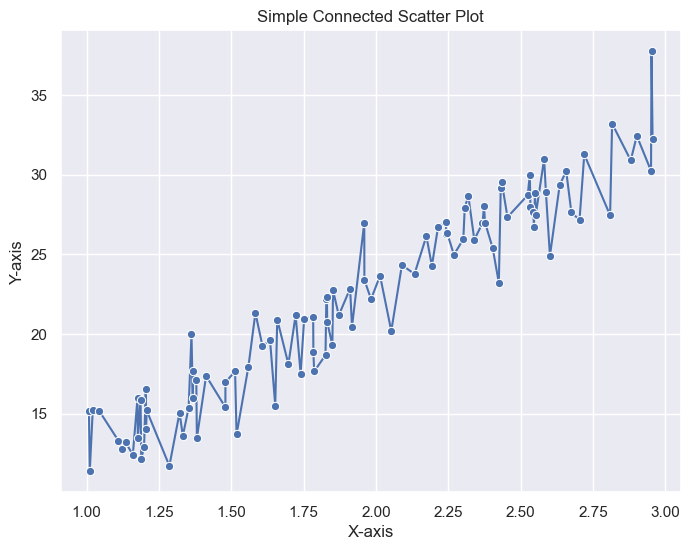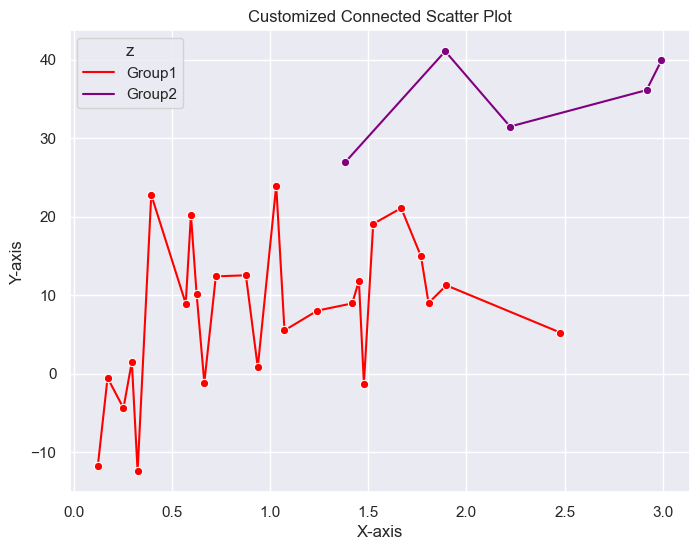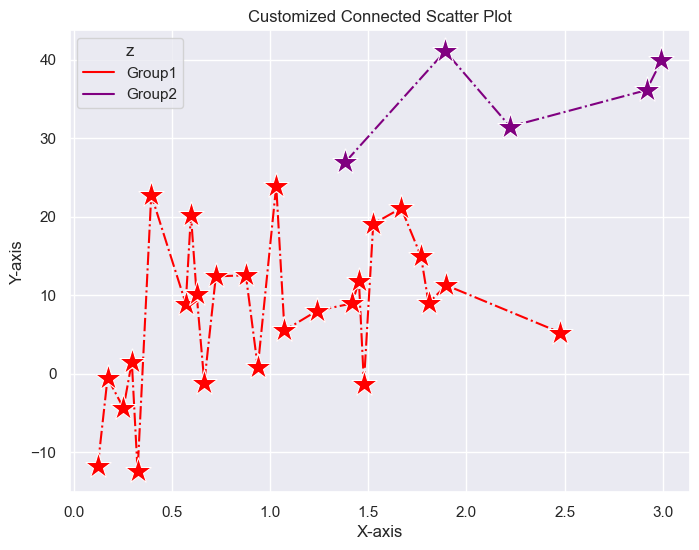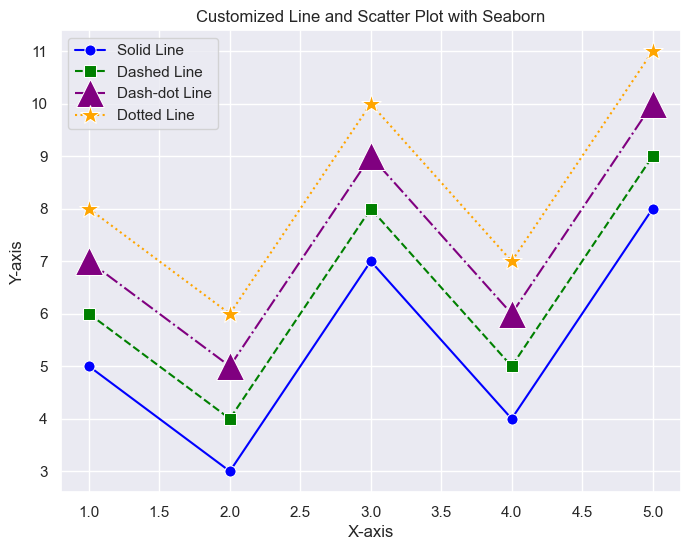### Simple scatter plot

A `simple scatter plot` is a graphical representation that displays individual data points as dots on a two-dimensional plane. Each dot represents a single observation or data point, and its position on the plot corresponds to the values of two variables.

### Connected scatter plot

A `connected scatter plot`, also known as a line plot with markers, is similar to a simple scatter plot but with the addition of lines connecting the data points in the order they appear. This type of plot is often used to visualize the temporal or sequential relationships between two variables.

## Libraries

First, you need to install the following librairies:

• seaborn is used for creating the chart witht the `lineplot()` function
• matplotlib is used for plot customization purposes
• `numpy` is used to generate some data
• `pandas` is used to store the data generated with numpy

Don't forget to install seaborn if you haven't already done so with the `pip install seaborn` command.

``````# Use the darkgrid theme for seaborn
import seaborn as sns
sns.set_theme(style="darkgrid")

import matplotlib.pyplot as plt
import numpy as np
import pandas as pd``````

## Dataset

For scatter plot, we need 2 numeric variables. We randomly generate these variables thanks to numpy's `random.uniform()` and `random.normal()` function that, respectively, generate uniformly and normally distributed data.

``````# Generate random numeric values, where y is a function of x
x = np.random.uniform(3,1,100)
y = x * 10 + np.random.normal(3,2,100)

data = {'x': x,
'y': y,
}
df = pd.DataFrame(data)``````

## Basic connected scatter plot

The following code displays a simple connected scatter plot, with a title and an axis name, thanks to the `lineplot()` function from Seaborn.

``````plt.figure(figsize=(8, 6)) # Width and Height of the chart
sns.lineplot(x='x',
y='y',
data=df,
marker='o', # Style used to mark the join between 2 points
)
plt.xlabel('X-axis') # x-axis name
plt.ylabel('Y-axis') # y-axis name
plt.title('Simple Connected Scatter Plot') # Add a title
plt.show() # Display the graph``````## Customization features

### Display multiple lines on the same graph

To our previous dataset, we add a 3rd variable which will be categorical, named `z`. It will be used to separate our observations according to their label in this variable. In practice, just add `hue='z'` (or the name of the variable you want to use to divide the rows) when using the `lineplot` function.

You can use the `palette` parameter to specify your own colors for lines. If you want a specific color for each group, I recommend using a dictionary with label as key and color as value (which is what we do in the following example). But it's also possible to make a list of colors (however, it must be the same length as the number of distinct labels in 'z').

``````# Generate random numeric values, where y is a function of x
x = np.random.uniform(3,0.1,30)
y = x * 10 + np.random.normal(3,10,30)
z = ['Group1' if i < 25 else 'Group2' for i in y] # Categorical variable

data = {'x': x,
'y': y,
'z': z,
}
df = pd.DataFrame(data)``````
``````plt.figure(figsize=(8, 6)) # Width and Height of the chart
sns.lineplot(x='x',
y='y',
hue='z', # Create 2 line plots according to labels in 'z'
data=df,
marker='o', # Style used to mark the join between 2 points
palette={'Group1':'red',
'Group2':'purple'}, # Colors of the lines
)
plt.xlabel('X-axis') # x-axis name
plt.ylabel('Y-axis') # y-axis name
plt.title('Customized Connected Scatter Plot') # Add a title
plt.show() # Display the graph``````## Customize lines and markers

You can also customize markers and lines, thanks to several arguments.

• `marker`: the style of the marker (must be in the following list: `o`, `s`, `d`, `^`, `v`, `<`, `>`, `p`, `*`, `+`, `x`, `h`)
• `markersize`: marker size
• `linestyle`: the style of the line (must be in the following list: `-`, `--`, `-.`, `:`, `None`)
``````plt.figure(figsize=(8, 6)) # Width and Height of the chart
sns.lineplot(x='x',
y='y',
hue='z', # Create 2 line plots according to labels in 'z'
data=df,
marker='*', # Style used to mark the join between 2 points
markersize=20, # Size of the marker
palette={'Group1':'red',
'Group2':'purple'}, # Colors of the lines,
linestyle='-.', # Style used for the lines
)
plt.xlabel('X-axis') # x-axis name
plt.ylabel('Y-axis') # y-axis name
plt.title('Customized Connected Scatter Plot') # Add a title
plt.show() # Display the graph``````## A complete example that covers all the previous concepts

To give you some ideas for customization, here's a complete example of a connected scatter plot using the same concepts as above.

``````# Sample data
x = [1, 2, 3, 4, 5]
y = [5, 3, 7, 4, 8]

# Set Seaborn style
plt.figure(figsize=(8, 6))

# Solid line with circle markers
sns.lineplot(x=x, y=y, linestyle='-', marker='o', markersize=8, label='Solid Line', color='blue')

# Dashed line with square markers
sns.lineplot(x=x, y=[i + 1 for i in y], linestyle='--', marker='s', markersize=8, label='Dashed Line', color='green')

# Dash-dot line with triangle up markers
sns.lineplot(x=x, y=[i + 2 for i in y], linestyle='-.', marker='^', markersize=20, label='Dash-dot Line', color='purple')

# Dotted line with asterisk markers
sns.lineplot(x=x, y=[i + 3 for i in y], linestyle=':', marker='*', markersize=15, label='Dotted Line', color='orange')

plt.title('Customized Line and Scatter Plot with Seaborn') # Add a title
plt.xlabel('X-axis') # x-axis name
plt.ylabel('Y-axis') # x-axis name
plt.legend(loc='upper left') # Add a legend
plt.show() # Display the graph``````## Going further

This post explained how to create a connected scatter plot with different customization features using Python and its Seaborn library.

For more examples of how to create or customize your scatter plots with Python, check the scatter plot section. You might be interested in how to make a scatter plot with a linear regression on it.

## Contact & Edit

👋 This document is a work by Yan Holtz. You can contribute on github, send me a feedback on twitter or subscribe to the newsletter to know when new examples are published! 🔥